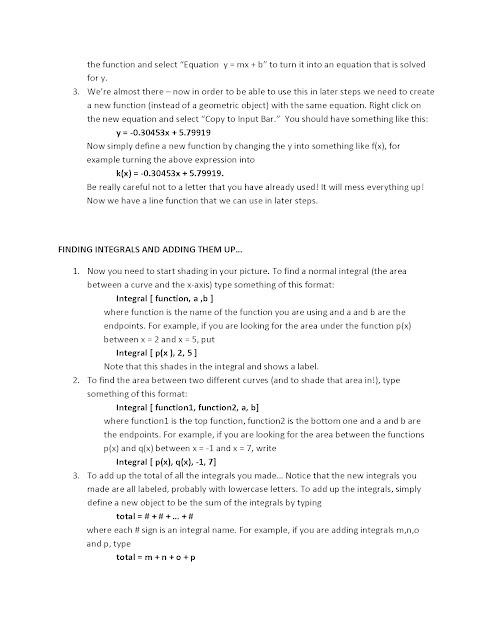## Wednesday, June 6, 2012

### Integration and Geogebra

I gave the following as my integration unit exam.  I have to thank Bowman Dickson @bowmanimal, his blog is http://bowmandickson.com/, for giving me the instructions and project with the geogebra.  Below is the assignment as well as some of the pictures I recieved.

Integration Project

1.      Create an equation for the velocity of a particle at any time t, stating the initial position, which cannot equal 0.  The equation must include all of:

·         A polynomial function

·         Rational function –Chain rule must be applied

·         Trigonometric function

a.       Determine, using appropriate sums of rectangles, an over and under estimation of the displacement of the particle in the first 10 seconds.

i.      Explain how this estimation could be made more exact.

b.      Determine the exact displacement of the particle for the first 10 seconds, and then determine the exact location of the particle after 10 seconds.

c.       Determine the average acceleration of the particle from 0.  Illustrate how your answer could have been determined by the graphs.

2.      Complete a picture using geogebra with at least 5 areas calculated by hand.
Examples of the pictures

And here is what one looks like after the lines are removed.

Instructions for Geogebra:1.Nice activity. Thanks for sharing.

2.Great activity and very clear geogebra instructions - excellent stuff!

3.Awesome. Using next year for sure!

4.what was the criterion on which they where graded for the second problem ?

5.This is a great assignment